# ICSE Class 10 Maths Question Paper Solution 2019

The best way to prepare for ICSE 10th board Mathematics exam is by solving the end number of questions. One of the crucial things that students should not miss while preparing for Maths exam is solving the ICSE Class 10 Maths Question Paper Solution 2019. So, to help them prepare effectively for the exam, we have provided the ICSE Class 10 Maths Question Paper 2019 along with the Solutions. The answers are provided in steps along with the marking scheme for easy understanding of the students.

The ICSE Class 10 Maths 2019 exam was conducted on 8th March 2019. The exam started at 11am and students were allotted 2 hours 30 minutes of time duration to finish the paper. Students can download the ICSE Class 10 Maths Question Paper Solution 2019 PDF from the link below.

Students can have a look at the ICSE Class 10 Maths Question Paper Solution 2019 below: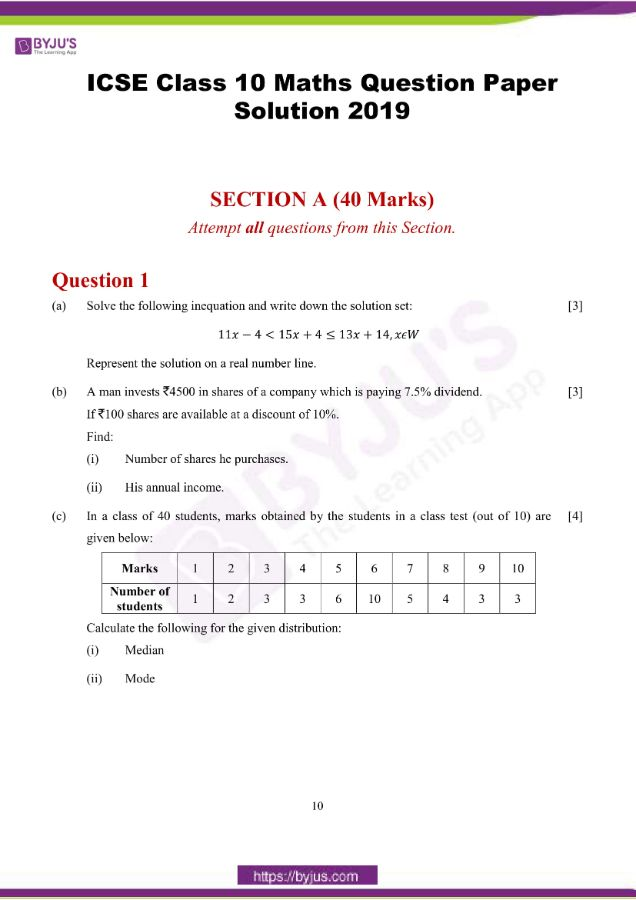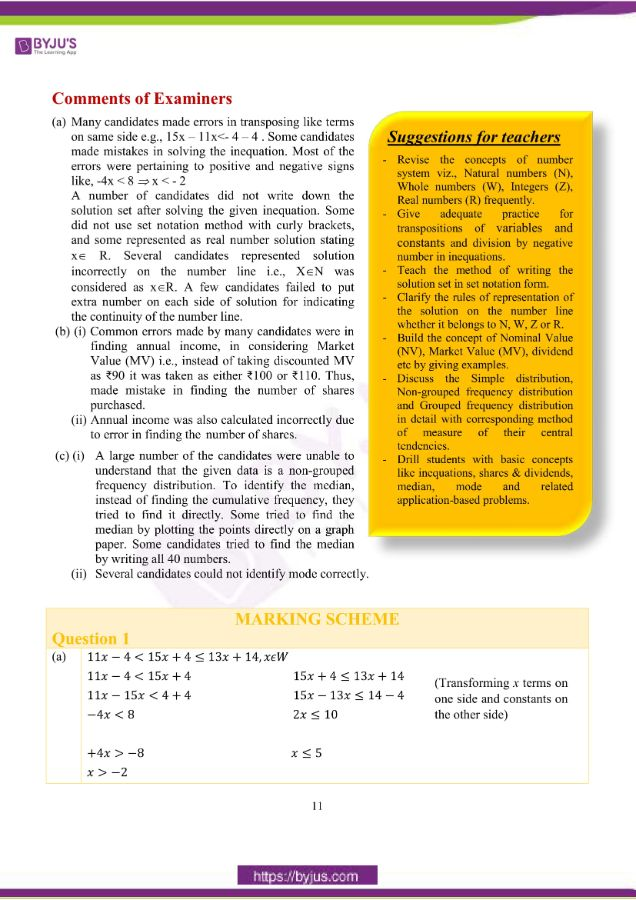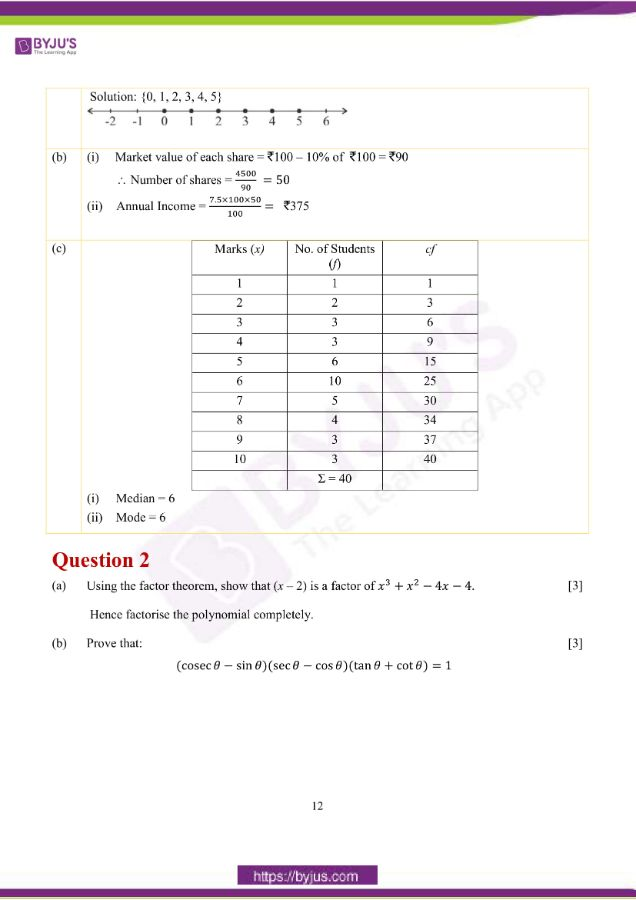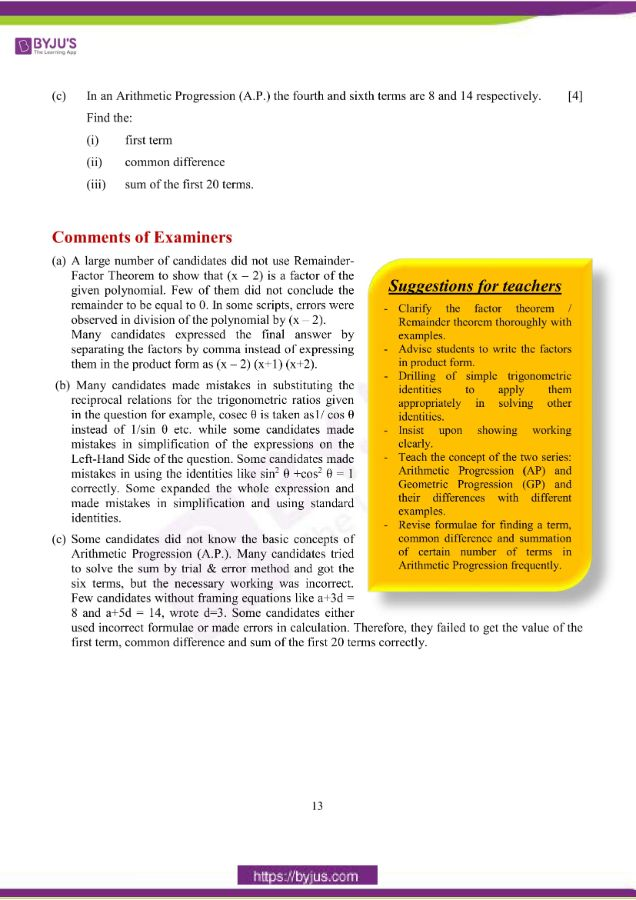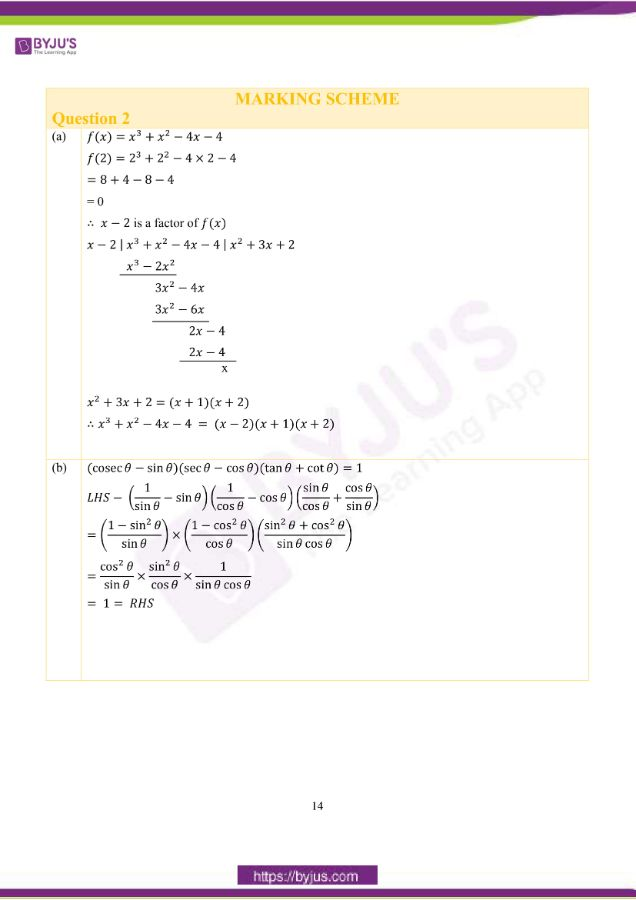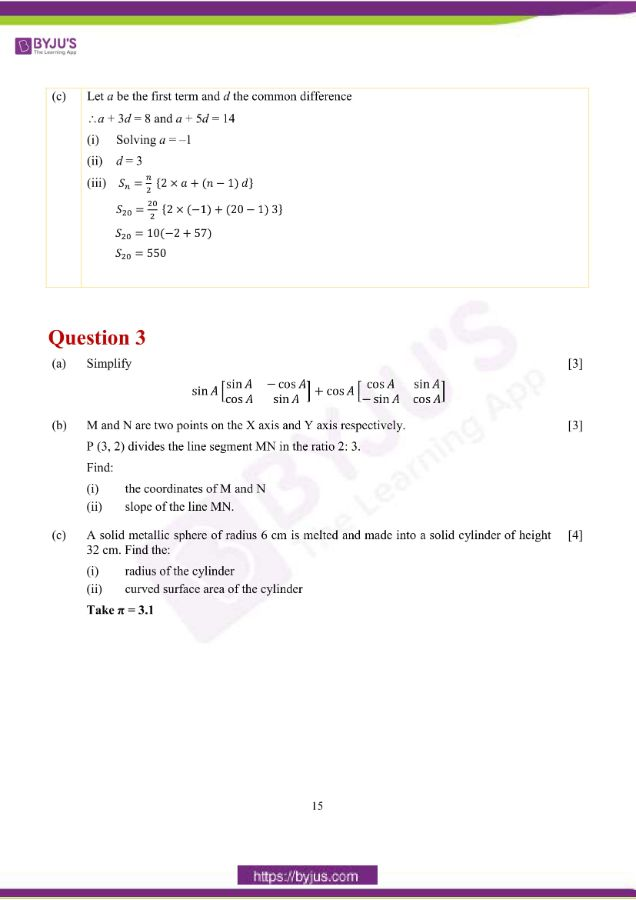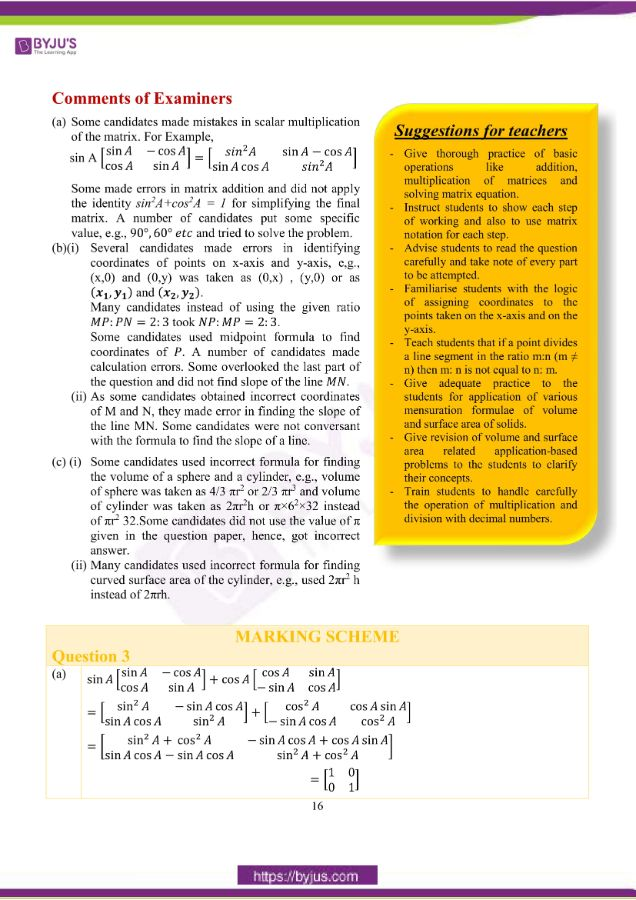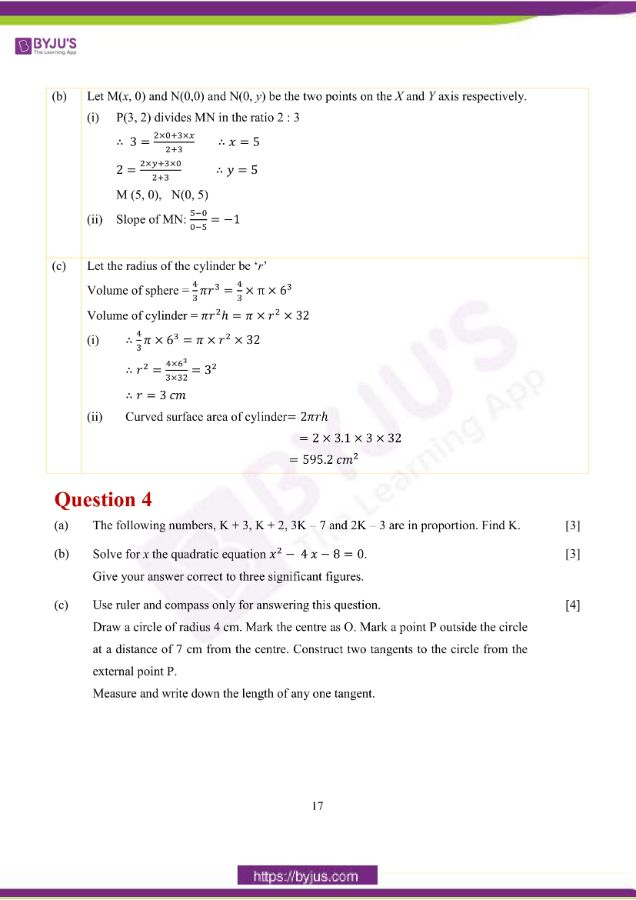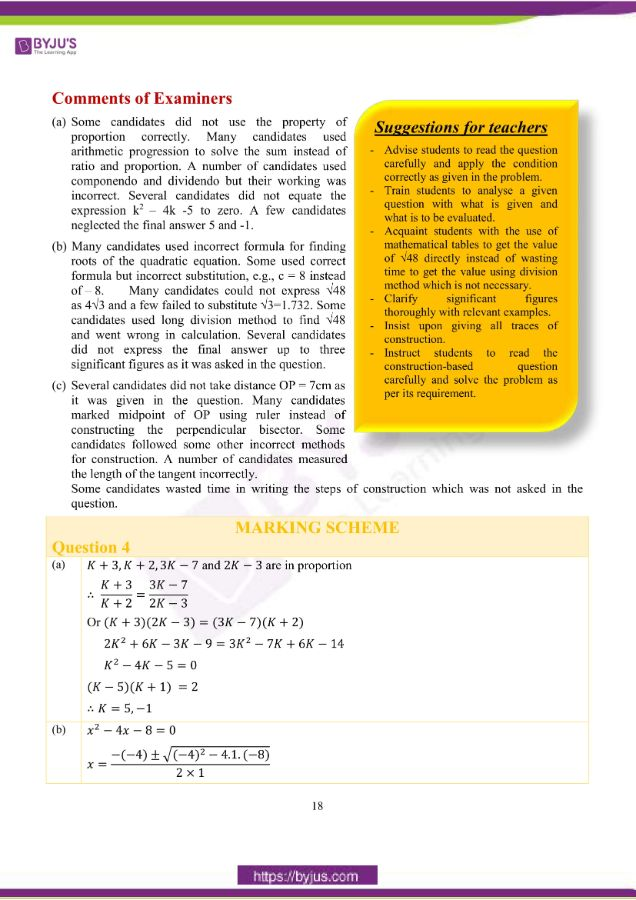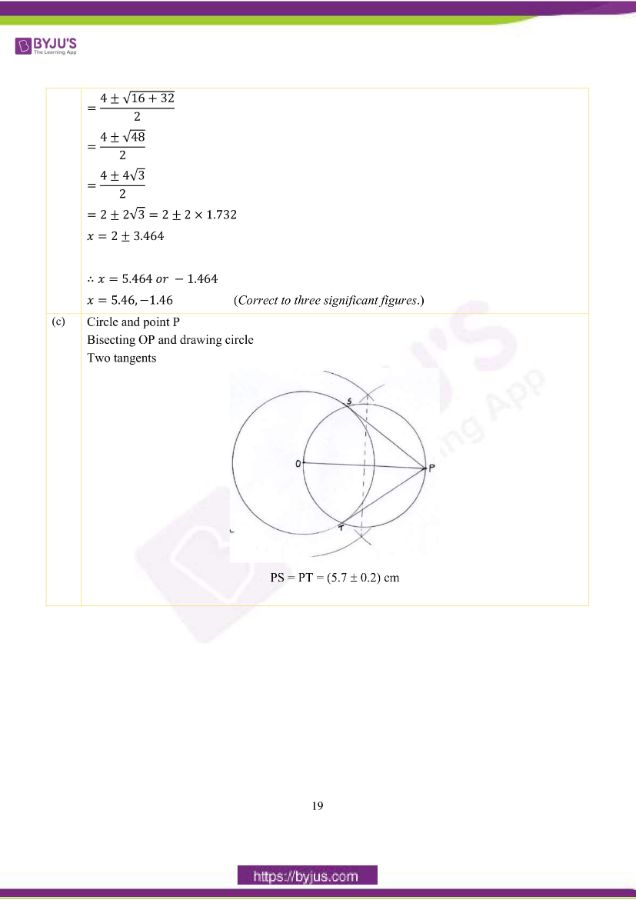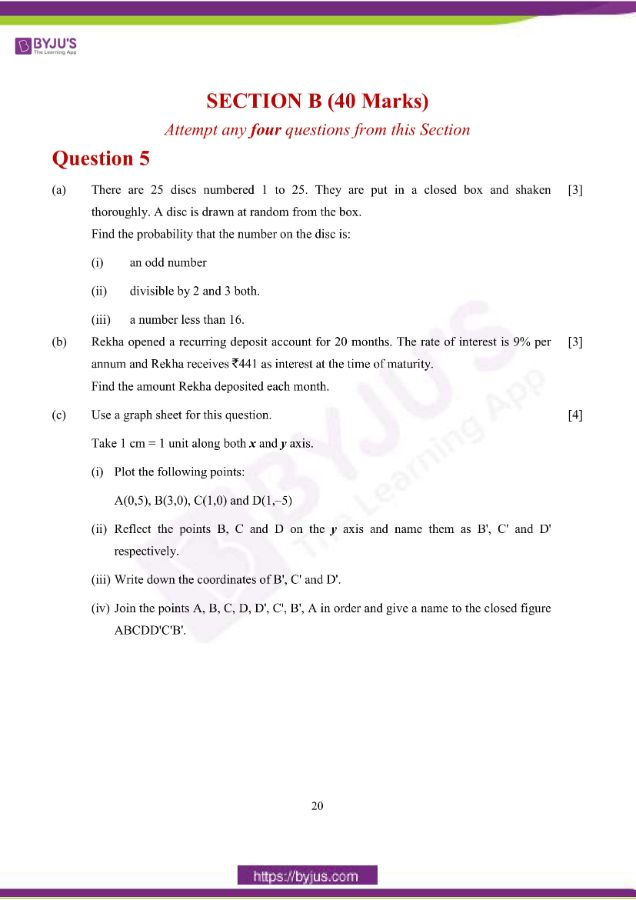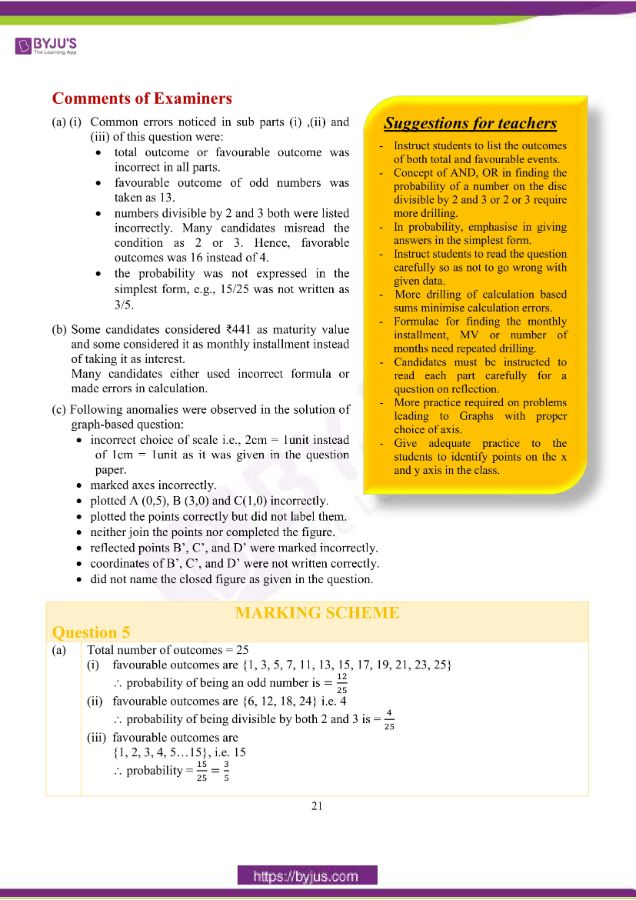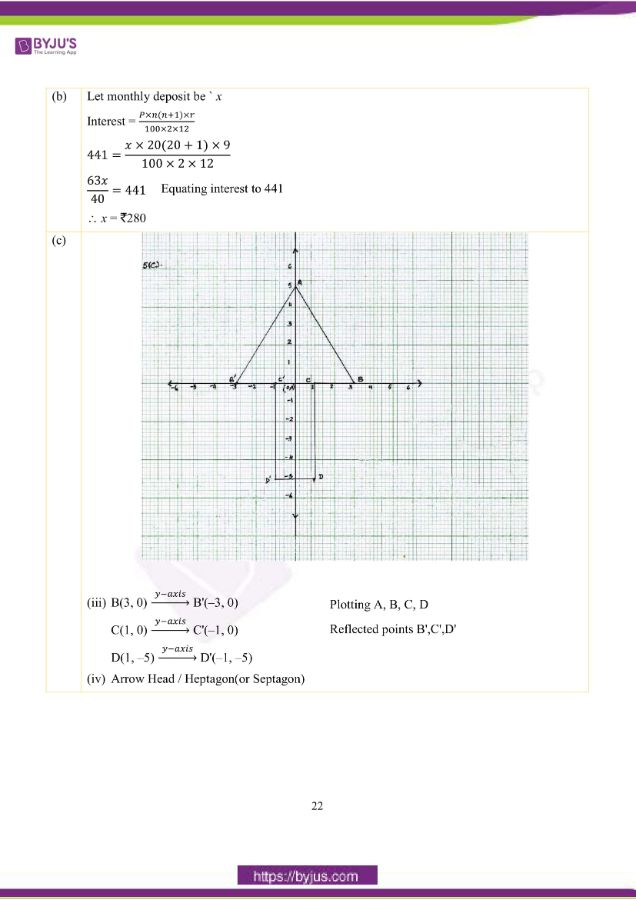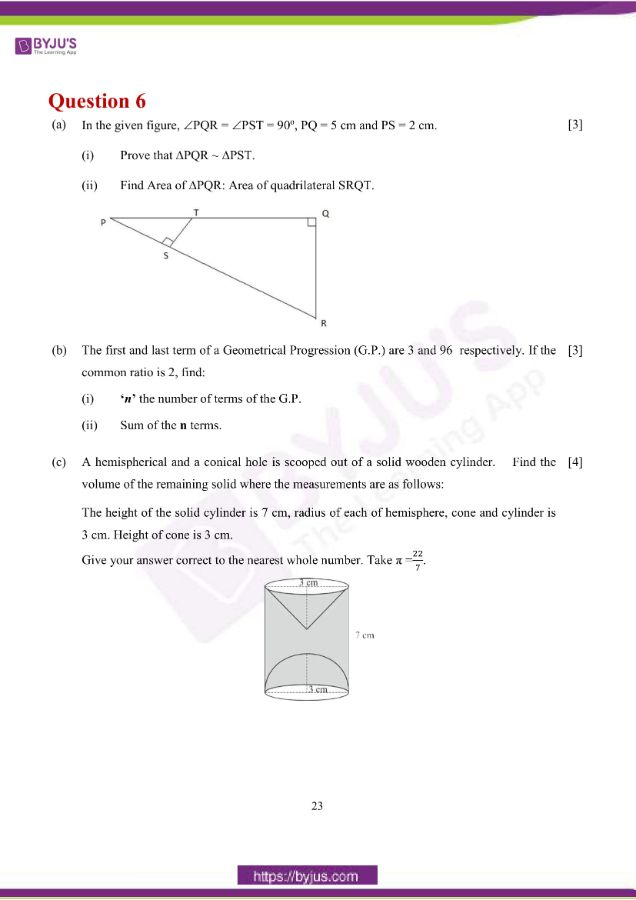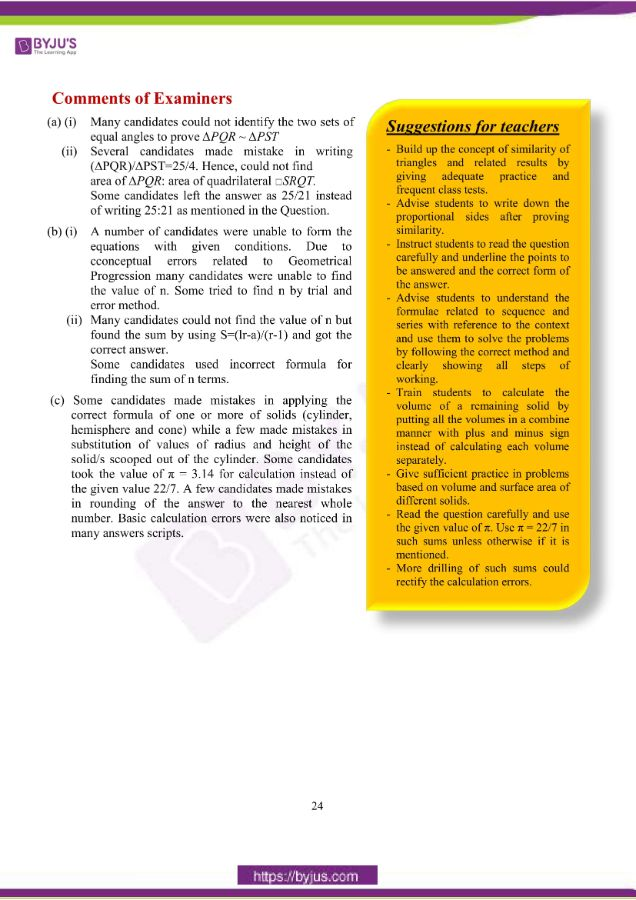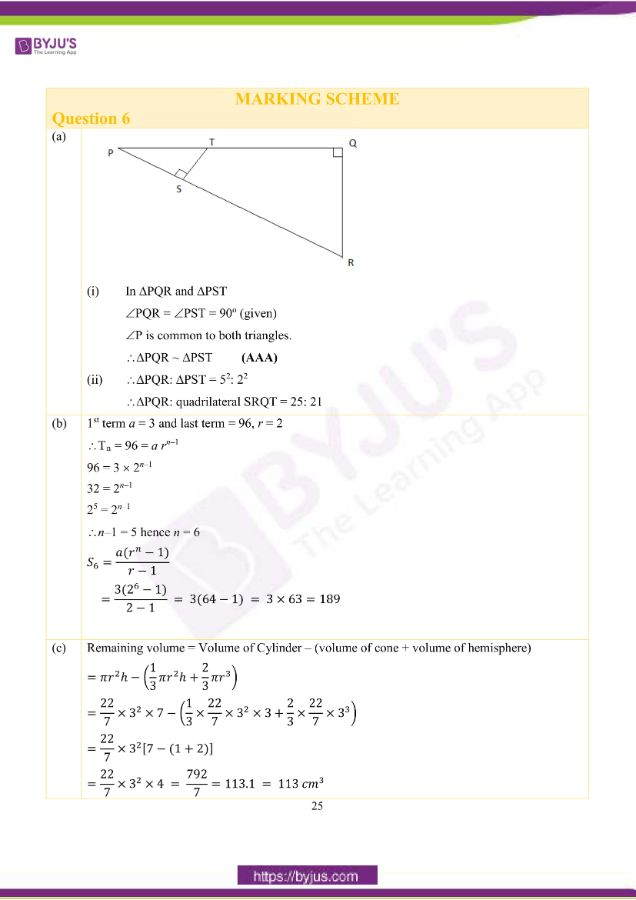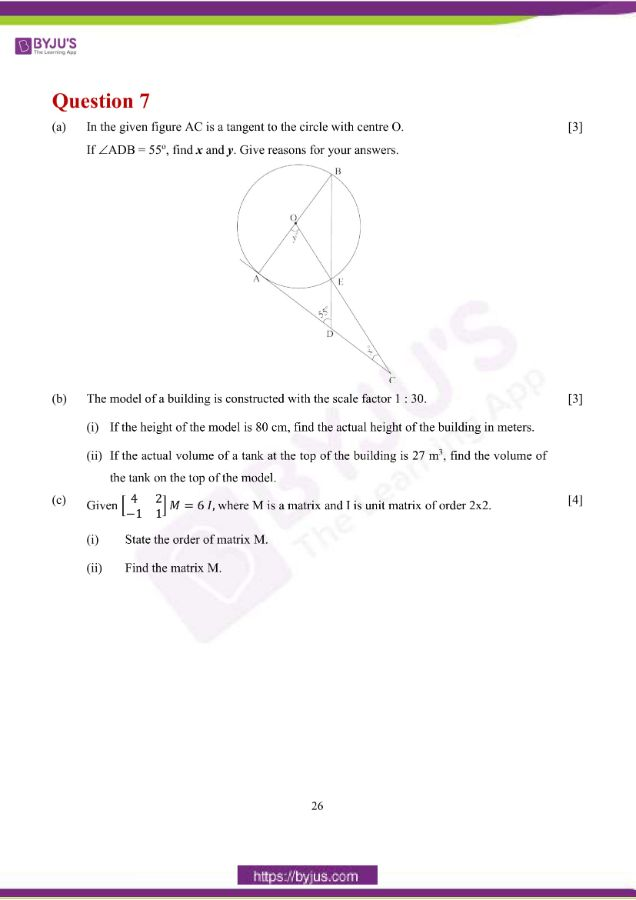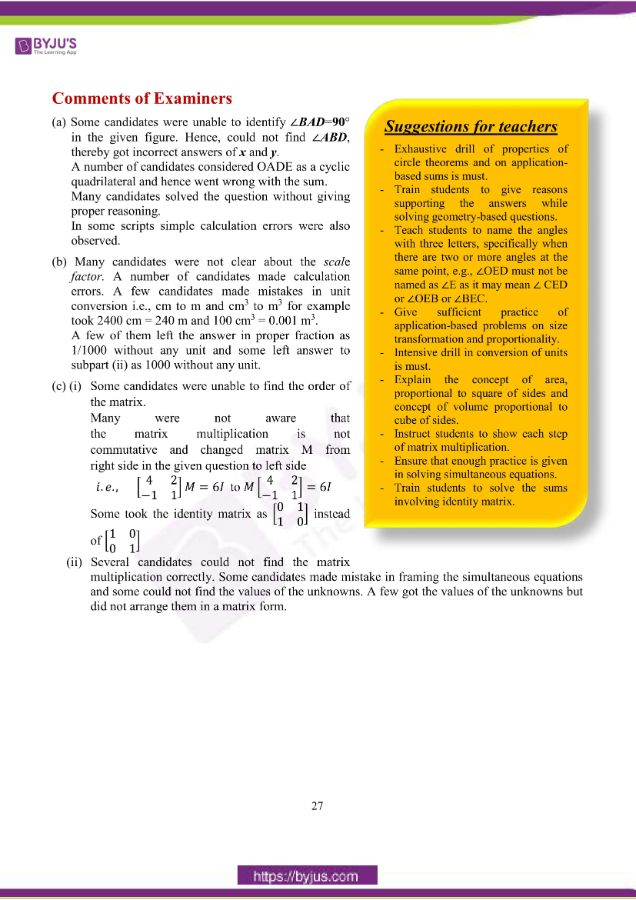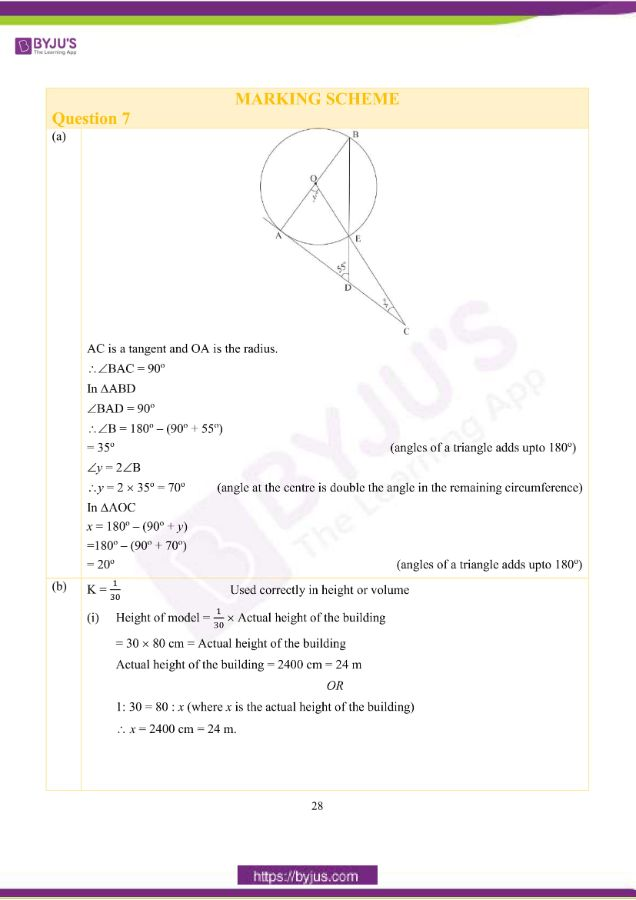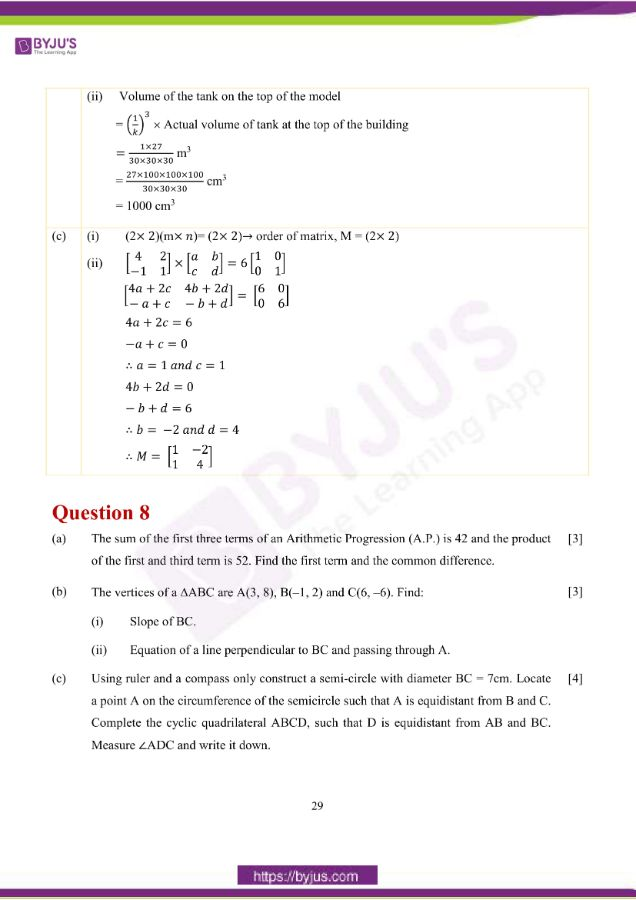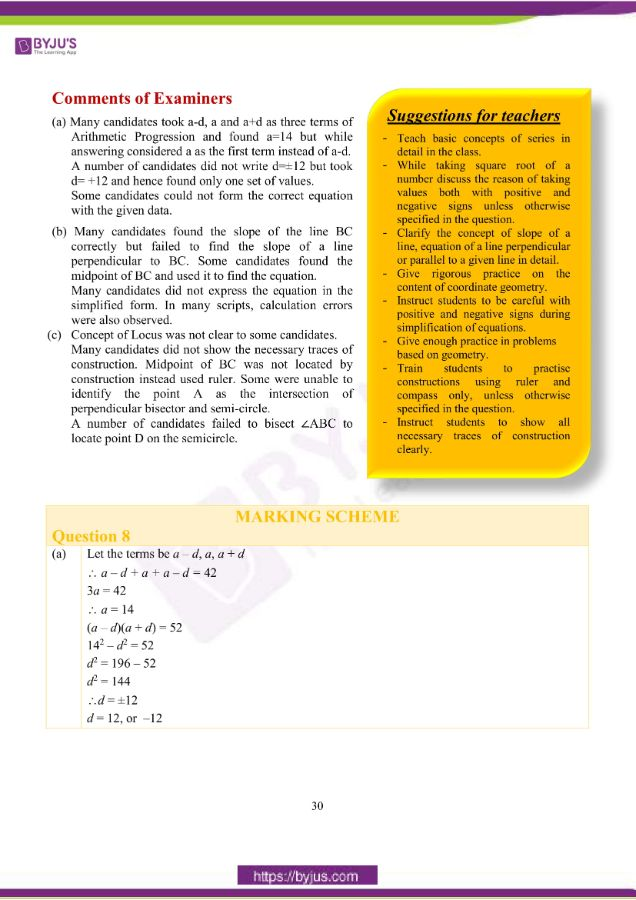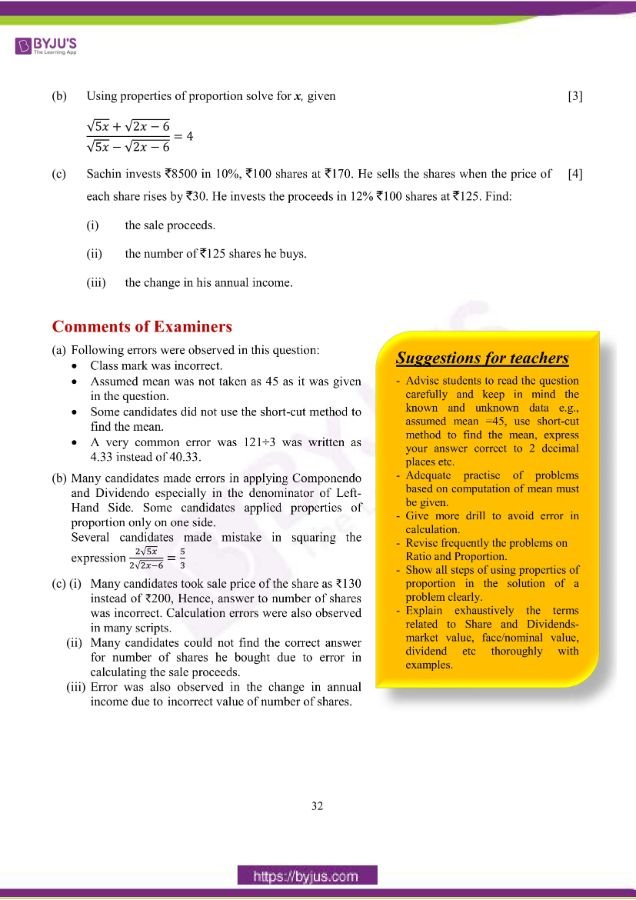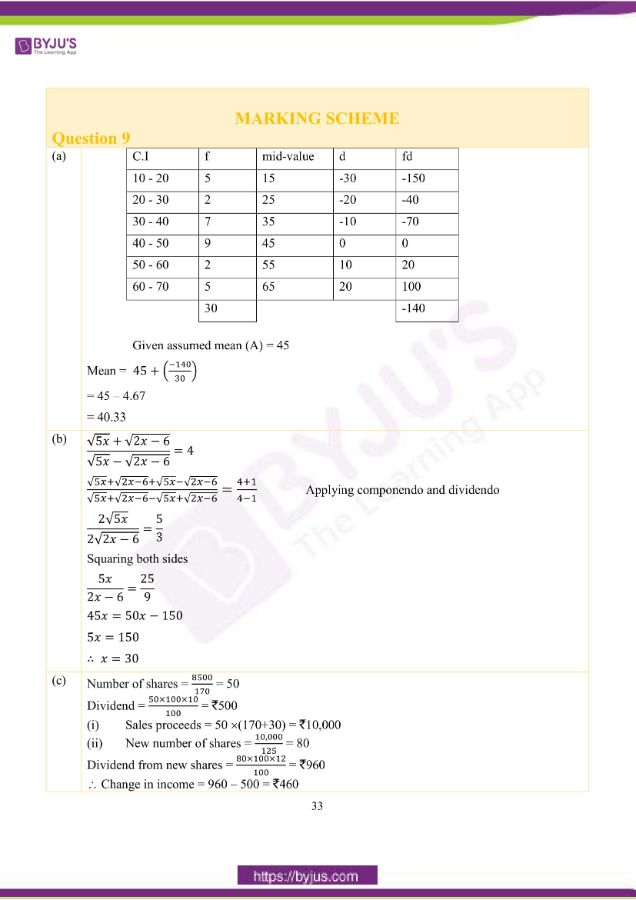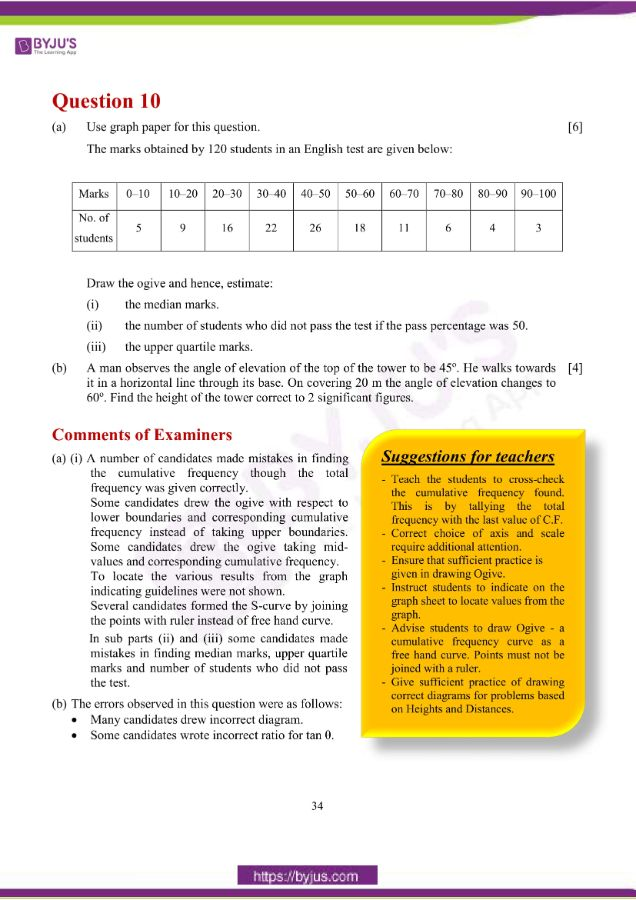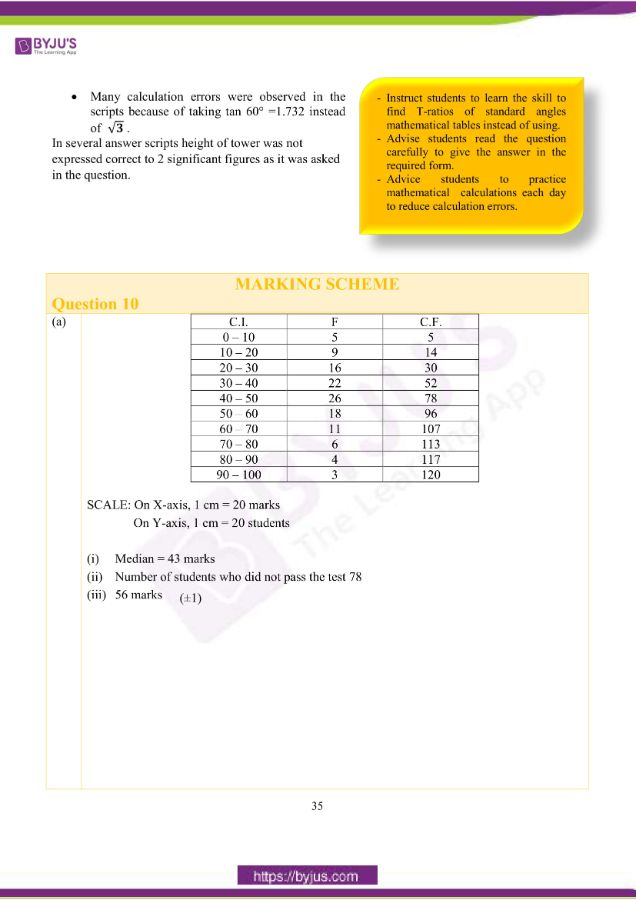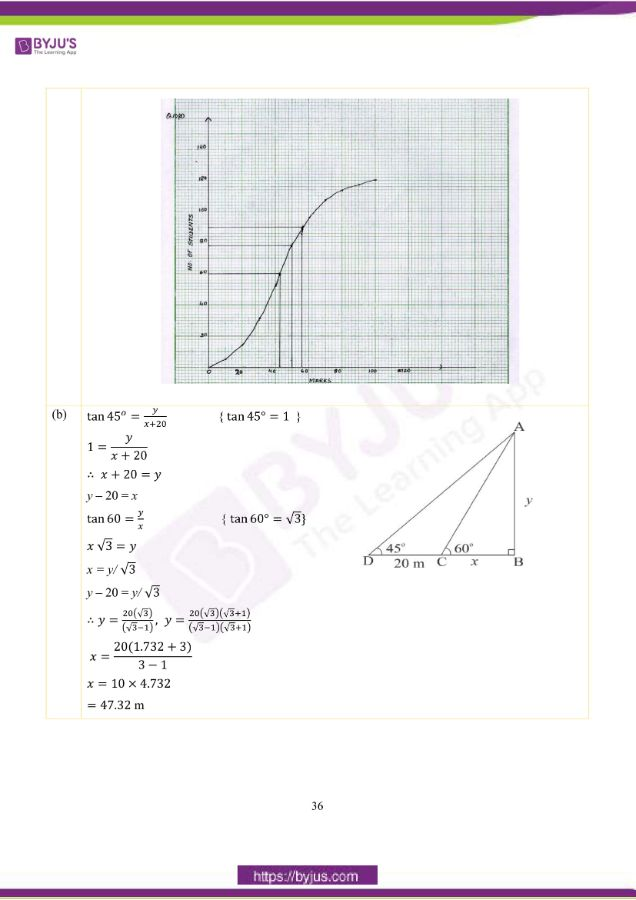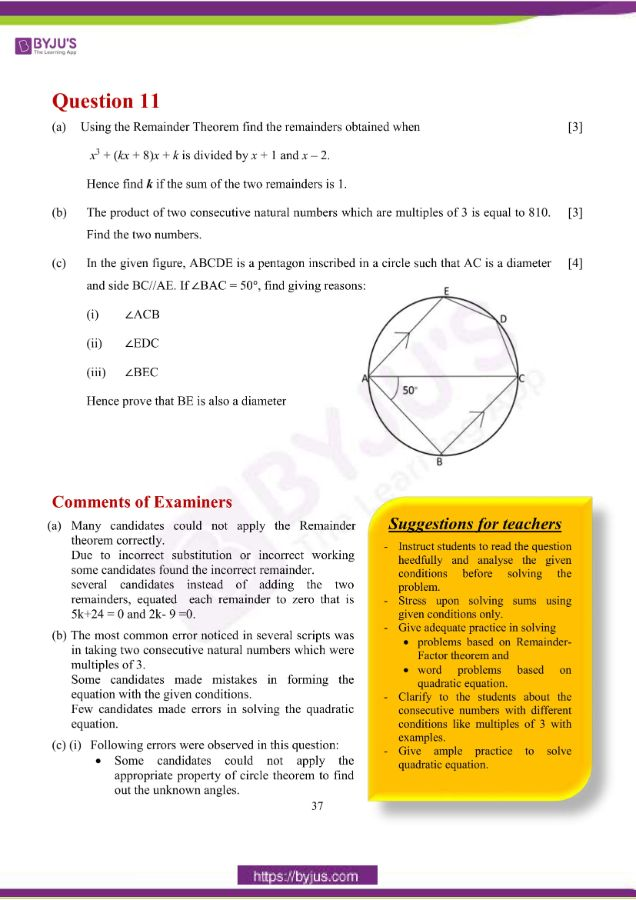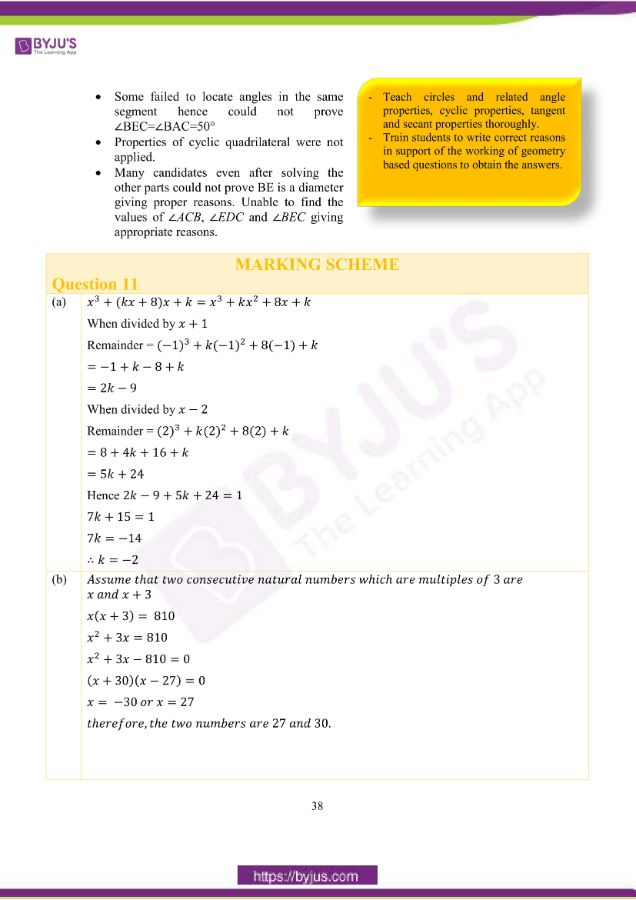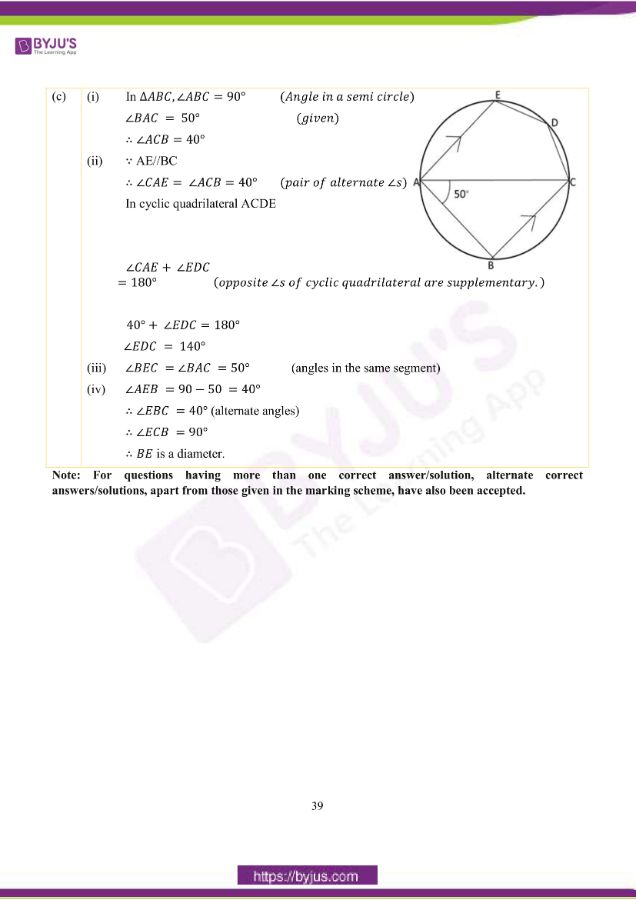## ICSE Class 10 Maths Question Paper 2019 With Solution

### Question 1:

(a) Solve the following inequation and write down the solution set:

11𝑥 − 4 < 15𝑥 + 4 ≤ 13𝑥 + 14, 𝑥⊂W

Represent the solution on a real number line.

(b) A man invests 4500 in shares of a company which is paying 7.5% dividend. If 100 shares are available at a discount of 10%.

Find:

(i) Number of shares he purchases.

(ii) His annual income.

(c) In a class of 40 students, marks obtained by the students in a class test (out of 10) are

given below:

 Marks 1 2 3 4 5 6 7 8 9 10 Number of students 1 2 3 3 6 10 5 4 3 3

Calculate the following for the given distribution:

(i) Median

(ii) Mode

(a)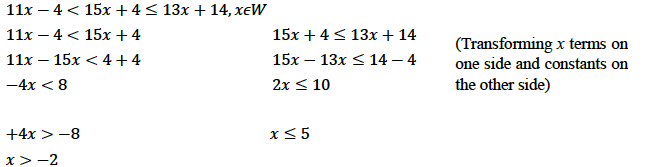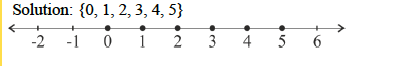(b) (i) Market value of each share = 100 – 10% of 100 = 90

∴ Number of shares = 4500/90 = 50

(ii) Annual Income = (7.5 × 100 × 50) / 100 = 375

(c)

 Marks (x) No. of Students (f) cf 1 1 1 2 2 3 3 3 6 4 3 9 5 6 15 6 10 25 7 5 30 8 4 34 9 3 37 10 3 40 Ʃ = 40

(i) Median = 6

(ii) Mode = 6

### Question 2:

(a) Using the factor theorem, show that (x – 2) is a factor of 𝑥3 + 𝑥2 − 4𝑥 − 4.

Hence factorise the polynomial completely.

(b) Prove that:

(cosec 𝜃 − sin 𝜃)(sec 𝜃 − cos 𝜃)(tan 𝜃 + cot 𝜃) = 1

(c) In an Arithmetic Progression (A.P.) the fourth and sixth terms are 8 and 14 respectively.

Find the:

(i) first term

(ii) common difference

(iii) sum of the first 20 terms.

(a)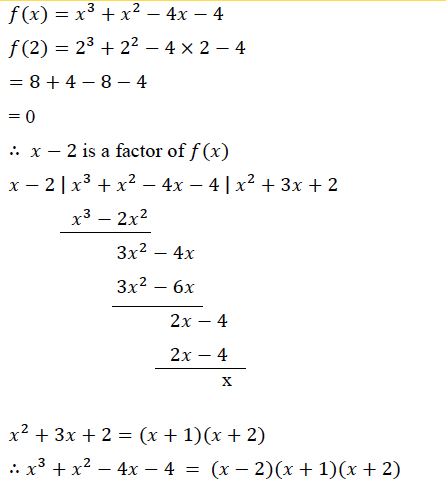(b)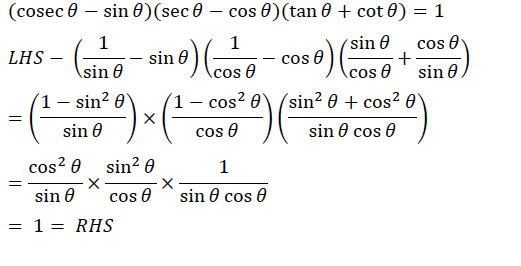(c) Let a be the first term and d the common difference

∴ a + 3d = 8 and a + 5d = 14

(i) Solving a = –1

(ii) d = 3

(iii) Sn = n/2 {2 × a + (n – 1) d}

S20 = 20/2 {2 × (-1) + (20 – 1) 3}

S20 = 10 (-2 + 57)

S20 = 550

### Question 3:

(a) Simplify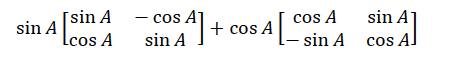(b) M and N are two points on the X axis and Y axis respectively. P (3, 2) divides the line segment MN in the ratio 2: 3.

Find:

(i) the coordinates of M and N

(ii) slope of the line MN.

(c) A solid metallic sphere of radius 6 cm is melted and made into a solid cylinder of height 32 cm.

Find the:

(ii) curved surface area of the cylinder

Take π = 3.1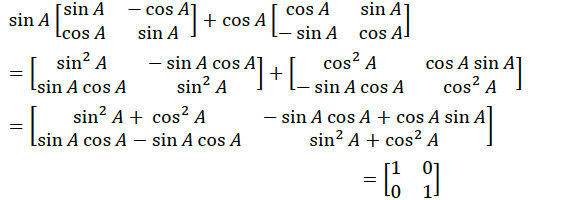(b) Let M(x, 0) and N(0,0) and N(0, y) be the two points on the X and Y axis respectively.

(i) P(3, 2) divides MN in the ratio 2 : 3

∴ 3 = (2×0 + 3×𝑥) / 2+3 ∴ 𝑥 = 5

2 = (2×𝑦 + 3×0)/ 2+3 ∴ 𝑦 = 5

M (5, 0), N(0, 5)

(ii) Slope of MN: (5−0)/(0−5) = −1

(c)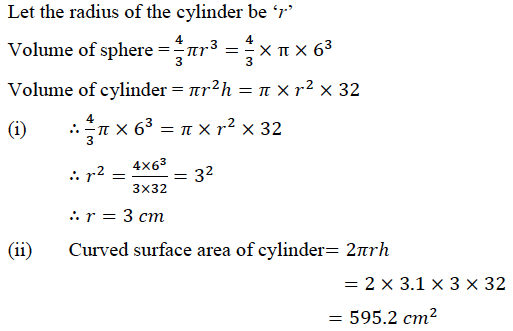### Question 4:

(a) The following numbers, K + 3, K + 2, 3K – 7 and 2K – 3 are in proportion. Find K.

(b) Solve for x the quadratic equation 𝑥2 − 4 𝑥 − 8 = 0.

(c) Use ruler and compass only for answering this question.

Draw a circle of radius 4 cm. Mark the centre as O. Mark a point P outside the circle at a distance of 7 cm from the centre. Construct two tangents to the circle from the external point P.

Measure and write down the length of any one tangent.

(a) 𝐾 + 3, 𝐾 + 2, 3𝐾 − 7 and 2𝐾 − 3 are in proportion

(𝐾 + 3)/(𝐾 + 2) = (3𝐾 − 7)/(2𝐾 − 3)

OR

(𝐾 + 3)(2𝐾 − 3) = (3𝐾 − 7)(𝐾 + 2)

2𝐾2 + 6𝐾 − 3𝐾 − 9 = 3𝐾2 − 7𝐾 + 6𝐾 − 14

𝐾2 − 4𝐾 − 5 = 0

(𝐾 − 5)(𝐾 + 1) = 2

∴ 𝐾 = 5, −1

(b)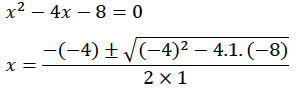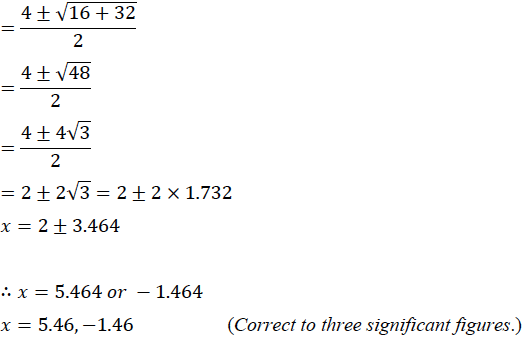(c) Circle and point P

Bisecting OP and drawing circle

Two tangents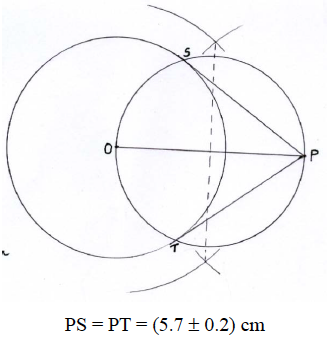### Question 5:

(a) There are 25 discs numbered 1 to 25. They are put in a closed box and shaken thoroughly. A disc is drawn at random from the box.

Find the probability that the number on the disc is:

(i) an odd number

(ii) divisible by 2 and 3 both.

(iii) a number less than 16.

(b) Rekha opened a recurring deposit account for 20 months. The rate of interest is 9% per annum and Rekha receives 441 as interest at the time of maturity.

Find the amount Rekha deposited each month.

(c) Use a graph sheet for this question.

Take 1 cm = 1 unit along both x and y axis.

(i) Plot the following points:

A(0,5), B(3,0), C(1,0) and D(1,–5)

(ii) Reflect the points B, C and D on the y axis and name them as B’, C’ and D’ respectively.

(iii) Write down the coordinates of B’, C’ and D’.

(iv) Join the points A, B, C, D, D’, C’, B’, A in order and give a name to the closed figure

ABCDD’C’B’.

(a) Total number of outcomes = 25

(i) favourable outcomes are {1, 3, 5, 7, 11, 13, 15, 17, 19, 21, 23, 25}

∴ probability of being an odd number is = 12/25

(ii) favourable outcomes are {6, 12, 18, 24} i.e. 4

∴ probability of being divisible by both 2 and 3 is = 4/25

(iii) favourable outcomes are

{1, 2, 3, 4, 5…15}, i.e. 15

∴ probability = 15/25 = 3/5

(b) Let monthly deposit be  x

Interest = {p × n(n+1) × r } / (100 × 2 × 12)

441 = {𝑥 × 20(20+1) × 9 } / (100 × 2 × 12)

63𝑥/40 = 441

∴ x = 280

(c)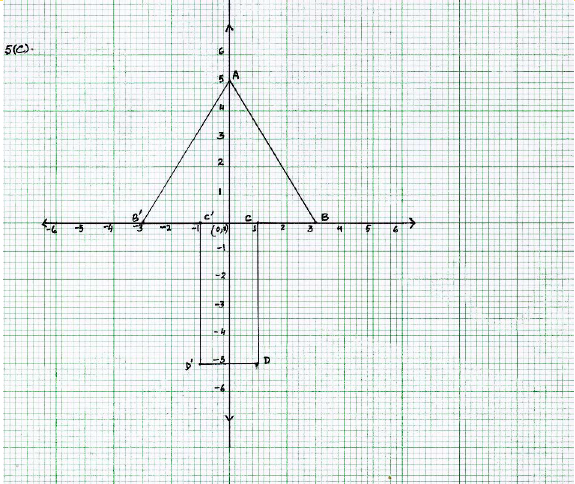(iii)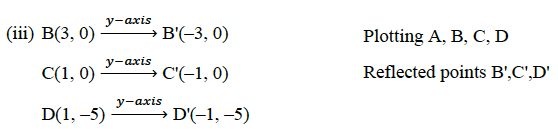(iv) Arrow Head / Heptagon(or Septagon)

### Question 6:

(a) In the given figure, ∠PQR = ∠PST = 90o, PQ = 5 cm and PS = 2 cm.

(i) Prove that ΔPQR ~ ΔPST.

(ii) Find Area of ΔPQR: Area of quadrilateral SRQT.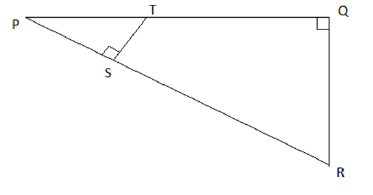(b) The first and last term of a Geometrical Progression (G.P.) are 3 and 96 respectively. If the common ratio is 2, find:

(i) ‘n’ the number of terms of the G.P.

(ii) Sum of the n terms.

(c) A hemispherical and a conical hole is scooped out of a solid wooden cylinder. Find the volume of the remaining solid where the measurements are as follows:

The height of the solid cylinder is 7 cm, radius of each of hemisphere, cone and cylinder is 3 cm. Height of the cone is 3 cm. Give your answer correct to the nearest whole number. Take π =22/7.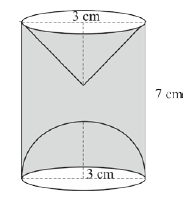(a)(i) In ΔPQR and ΔPST

∠PQR = ∠PST = 90o (given)

∠P is common to both triangles.

∴ ΔPQR ~ ΔPST (AAA)

(ii) ∴ ΔPQR: ΔPST = 52: 22

∴ ΔPQR: quadrilateral SRQT = 25: 21

(b) 1st term a = 3 and last term = 96, r = 2

∴Tn = 96 = a rn–1

96 = 3 × 2n–1

32 = 2n–1

25 = 2n–1

∴n–1 = 5 hence n = 6

S6 = a (rn – 1)/ r – 1

S6 = 3 (26 – 1)/ 2 – 1

S6 = 3 (64 − 1) = 3 × 63 = 189

(c)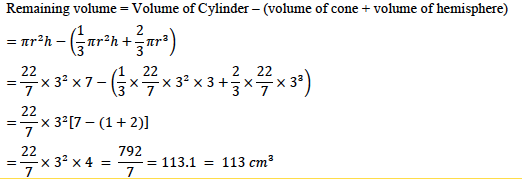### Question 7:

(a) In the given figure AC is a tangent to the circle with centre O.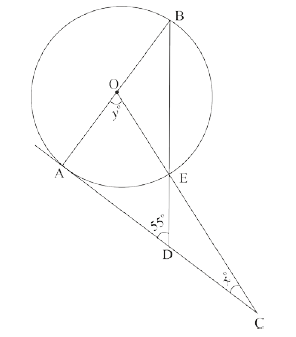(b) The model of a building is constructed with the scale factor 1 : 30.

(i) If the height of the model is 80 cm, find the actual height of the building in meters.

(ii) If the actual volume of a tank at the top of the building is 27 m3, find the volume of

the tank on the top of the model.

(c)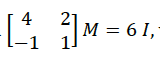where M is a matrix and I is unit matrix of order 2×2.

(i) State the order of matrix M.

(ii) Find the matrix M.

(a)AC is a tangent and OA is the radius.

∴∠BAC = 90o

In ΔABD

∴∠B = 180o – (90o + 55o)

= 35o (angles of a triangle adds upto 180o)

∠y = 2∠B

∴y = 2 × 35o = 70o (angle at the centre is double the angle in the remaining circumference)

In ΔAOC

x = 180o – (90o + y)

=180o – (90o + 70o)

= 20o (angles of a triangle adds upto 180o)

(b)

K = 1/30, Used correctly in height or volume

(i) Height of model = 1/30 × Actual height of the building

= 30 × 80 cm = Actual height of the building

Actual height of the building = 2400 cm = 24 m

OR

1: 30 = 80 : x (where x is the actual height of the building)

∴ x = 2400 cm = 24 m.

(ii) Volume of the tank on the top of the model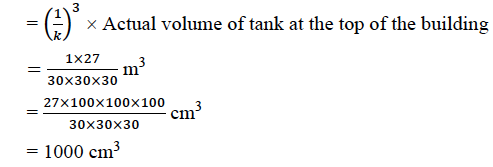(c) (i) (2 × 2)(m × 𝑛)= (2 × 2) → order of matrix, M = (2 × 2)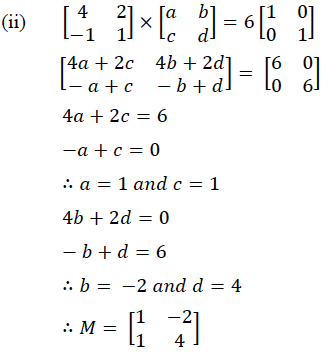### Question 8:

(a) The sum of the first three terms of an Arithmetic Progression (A.P.) is 42 and the product of the first and third term is 52. Find the first term and the common difference.

(b) The vertices of a ΔABC are A(3, 8), B(–1, 2) and C(6, –6).

Find:

(i) Slope of BC.

(ii) Equation of a line perpendicular to BC and passing through A.

(c) Using ruler and a compass only construct a semi-circle with diameter BC = 7cm. Locate a point A on the circumference of the semicircle such that A is equidistant from B and C. Complete the cyclic quadrilateral ABCD, such that D is equidistant from AB and BC. Measure ∠ADC and write it down.

(a) Let the terms be a – d, a, a + d

∴ a – d + a + a – d = 42

3a = 42

∴ a = 14

(a – d)(a + d) = 52

142 – d2 = 52

d2 = 196 – 52

d2 = 144

∴d = ±12

d = 12, or –12

(b) A(3, 8), B(–1, 2) and C(6, –6)

(i) Slope of line BC = (−6−2)/(6+1) = −8/7

(ii) Slope of line perpendicular to BC is 7/8; Line passing through A(3, 8)

Equation is:

𝑦 −8 = 7/8 (𝑥 − 3)

8𝑦 − 64 = 7𝑥 − 21

7𝑥 − 8𝑦 + 43 = 0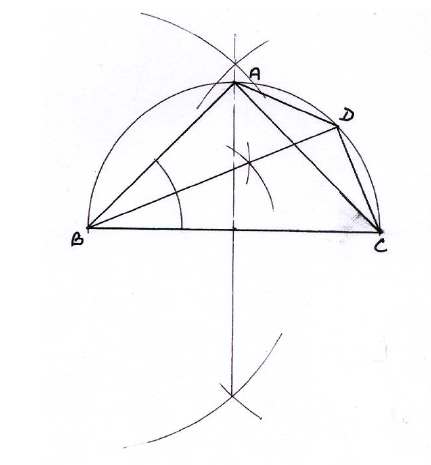### Question 9:

(a) The data on the number of patients attending a hospital in a month are given below. Find the average (mean) number of patients attending the hospital in a month by using the shortcut method.

Take the assumed mean as 45. Give your answer correct to 2 decimal places.

 Number of patients 10-20 20-30 30-40 40-50 50-60 60-70 Number of Days 5 2 7 9 2 5

(b) Using properties of proportion solve for x, given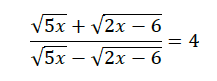(c) Sachin invests 8500 in 10%, 100 shares at 170. He sells the shares when the price of each share rises by 30. He invests the proceeds in 12% Rs. 100 shares at 125. Find:

(i) the sale proceeds

(ii) the number of 125 shares he buys

(iii) the change in his annual income

(a)

 C.I f mid-value d fd 10-20 5 15 -30 -150 20-30 2 25 -20 -40 30-40 7 35 -10 -70 40-50 9 45 0 0 50-60 2 55 10 20 60-70 5 65 20 100 30 -140

Given assumed mean (A) = 45

Mean = 45 + (-140/30)

= 45 – 4.67

= 40.33

(b)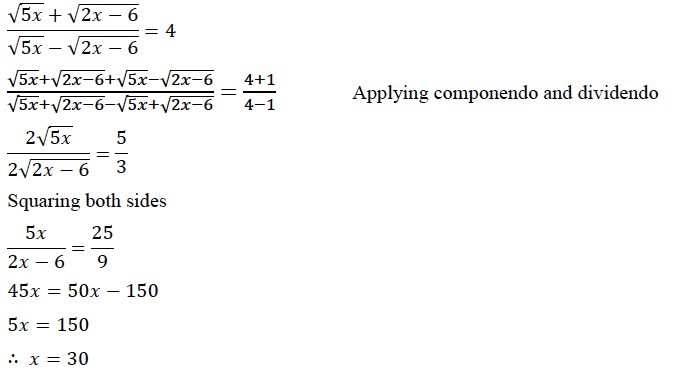(c) Number of shares = 8500/170 = 50

Dividend = (50 × 100 × 10)/ 100 = 500

(i) Sales proceeds = 50 ×(170+30) = 10,000

(ii) New number of shares = 10,000/125 = 80

Dividend from new shares = (80 × 100 × 12)/100 = 960

∴ Change in income = 960 – 500 = 460

### Question 10:

(a) Use graph paper for this question.

The marks obtained by 120 students in an English test are given below:

 Marks 0-10 10-20 20-30 30-40 40-50 50-60 60-70 70-80 80-90 90-100 No. of students 5 9 16 22 26 18 11 6 4 3

Draw the ogive and hence, estimate:

(i) the median marks.

(ii) the number of students who did not pass the test if the pass percentage was 50.

(iii) the upper quartile marks.

(b) A man observes the angle of elevation of the top of the tower to be 45o. He walks towards it in a horizontal line through its base. On covering 20 m the angle of elevation changes to 60o. Find the height of the tower correct to 2 significant figures.

(a)

 C.I F C.F 0-10 5 5 10-20 9 14 20-30 16 30 30-40 22 52 40-50 26 78 50-60 18 96 60-70 11 107 70-80 6 113 80-90 4 117 90-100 3 120

SCALE: On X-axis, 1 cm = 20 marks

On Y-axis, 1 cm = 20 students

(i) Median = 43 marks

(ii) Number of students who did not pass the test 78

(iii) 56 marks (±1)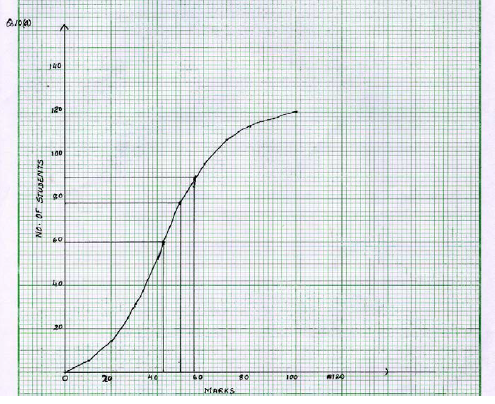(b)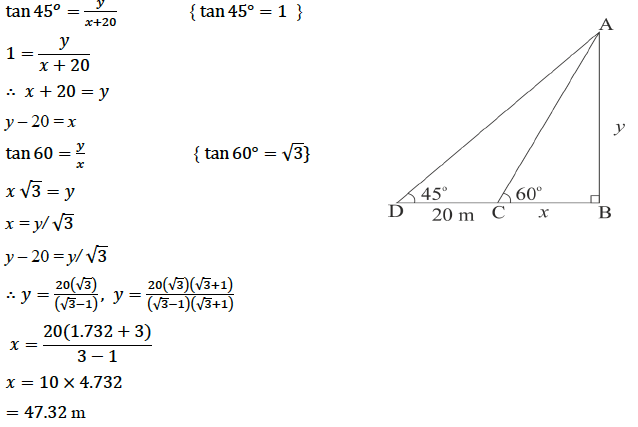### Question 11:

(a) Using the Remainder Theorem find the remainders obtained when

x3 + (kx + 8)x + k is divided by x + 1 and x – 2.

Hence find k if the sum of the two remainders is 1.

(b) The product of two consecutive natural numbers which are multiples of 3 is equal to 810. Find the two numbers.

(c) In the given figure, ABCDE is a pentagon inscribed in a circle such that AC is a diameter and side BC//AE. If ∠BAC = 500, find giving reasons:

(i) ∠ACB

(ii) ∠EDC

(iii) ∠BEC

Hence prove that BE is also a diameter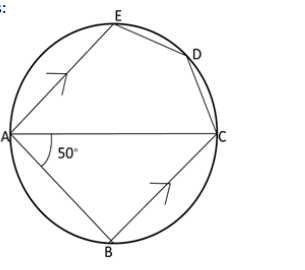(a) 𝑥3 + (𝑘𝑥 + 8)𝑥 + 𝑘 = 𝑥3 + 𝑘𝑥2 + 8𝑥 + 𝑘𝑘

When divided by 𝑥 + 1

Remainder = (−1)3 + 𝑘(−1)2 + 8(−1) + 𝑘

= −1 + 𝑘 − 8 +𝑘

= 2𝑘 − 9

When divided by 𝑥 − 2

Remainder = (2)3 + 𝑘(2)2 + 8(2)+𝑘

= 8+4𝑘 + 16 + 𝑘

= 5𝑘𝑘 + 24

Hence 2𝑘 − 9 + 5𝑘 + 24 = 1

7𝑘 + 15 = 1

7𝑘 = −14

∴ 𝑘 = −2

(b) Assume that two consecutive natural numbers which are multiple of 3 are

𝑥 𝑎𝑛𝑑 𝑥 + 3

𝑥(𝑥 + 3) = 810

𝑥2 + 3𝑥 = 810

𝑥2 + 3𝑥 − 810 = 0

(𝑥 + 30)(𝑥 − 27) = 0

𝑥 = −30 𝑜𝑟 𝑥= 27

Therefore, the two numbers are 27 and 30.

(c) (i) In ΔABC and ∠ABC = 900 (Angle is a semi circle)

∠BAC = 50° (given)

∴ ∠ACB = 40°

(ii) ∵ AE//BC

∴ ∠CAE = ∠𝐴CB = 40° (pair of alternate ∠s)

∠CAE + ∠EDC

= 180° (opposite ∠𝑠 𝑜𝑓 cyclic quadrilateral are supplementary)

40° + ∠𝐸DC = 180°

∠EDC = 140°

(iii) ∠BEC = ∠BAC = 50° (angles in the same segment)

(iv) ∠𝐴EB = 90 − 50 = 40°

∴ ∠EBC = 40° (alternate angles)

∴ ∠ECB = 90°

∴ BE is a diameter.

We hope this information on “ICSE Class 10 Maths Question Papers Solutions 2019” helped students in their exam preparation. To get the year wise ICSE Class 10 Previous Years Question papers along with solutions for other subjects, click here. Keep learning and download BYJU’S App to access interactive study videos.